# (Sample) Transfer Function Analysis and Design Tool - Result -

Calculated the Transfer Function, displayed on graphs, showing Bode diagram, Nyquist diagram, Impulse response and Step response.

## Transfer Function Analysis

(Sample) Transfer Function:
 G(s)= s+10000 s3+30s2+500s+10000

#### Pole(s)

p = -2.1552679847381 +19.611717445798i
|p|= 3.1400937168562[Hz]
p = -25.689464030524
|p|= 4.0886051858393[Hz]
p = -2.1552679847381-19.611717445798i
|p|= 3.1400937168562[Hz]

#### Zero(s)

z = -10000
|z|= 1591.549430919[Hz]#### Phase margin

pm= -21[deg] (f =4[Hz])

#### Oscillation frequency:

f = 3.1213017740203[Hz]

#### Overshoot (in absolute value)

The 1st peak  gpk = 1.55 (t =0.2[sec])
The 2nd peak  gpk = 0.61 (t =0.36[sec])
The 3rd peak  gpk = 1.28 (t =0.52[sec])

g(∞) = 1

β=
α=

### Frequency analysis

Bode diagram
Phase  Group delay
Nyquist diagram
Pole, zero
Phase margin
Oscillation analysis

Upper and lower frequency limits:
f1= - f2=[Hz] (frequency limits are optional)

### Transient analysis

Step response
Impulse response
Overshoot
Final value of the step response

Simulation time:
0〜[sec] (optional)

Transfer Function Design Tools ()

The form of α and β values is rewritten by this tool to design the transfer function at given some paramaters.

### Order poles on s-domain

Poles are ordered on s-domain of the transfer function inputted form of α and β. G(s) is rewritten that it solve the following equation.
G(s) = {the transfer function of inputted old α and β}×H(s)

• 1st order system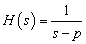p=
• 2nd order system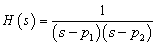p1,p2=±j
p1,p2=exp(±j )

### Order zeros on s-domain

The system of H(s) is setted zeros z, z1 and z2 of a given the following form. The matrix of α and β as transfer function G(s) is rewritten to add zeros. The G(s) is solved the following equation.
G(s) = {the transfer function of inputted old α and β}×H(s)
If α and β was blank, G(s) = H(s).

• 1st order system
H(s)= s - z    z=
• 2nd order system
H(s)=(s - z1 )(s - z2 )
z1,z2= ± j
z1,z2=exp(±j )

### Design from ζ and ω0 on a 2nd order system

Poles are ordered on s-domain of the transfer function inputted form of α and β. G(s) is rewritten that it solve the following equation.
G(s) = {the transfer function of inputted old α and β}×H(s)
If α and β was blank, G(s) = H(s).

2nd order system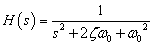•Damping ratio ζ=

### Design the coefficient of s2, s1, s0

G(s) is rewritten that it solve the following equation.
G(s) = {the transfer function of inputted old α and β}×H(s)
If α and β was blank, G(s) = H(s).

the transfer function
H(s)=β1s22s3

β1=0  β1=1  β1=

β2=0  β2=2ζω0 β2=
•Use the above form to input of ω0, ζ

β3=0  β302  β3=
•Use the above form to input of ω0

### Convert frequency→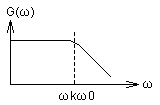ω0(natural angular frequency) from the transfer function of inputted α and β is converted that ω0 is multiplied by a given ωk.

ωk=

### Convert gain

G(s) transfer function of inputted α and β is multiplied by a given K.
G(s)←KG(s)

K=

# Frequency analysis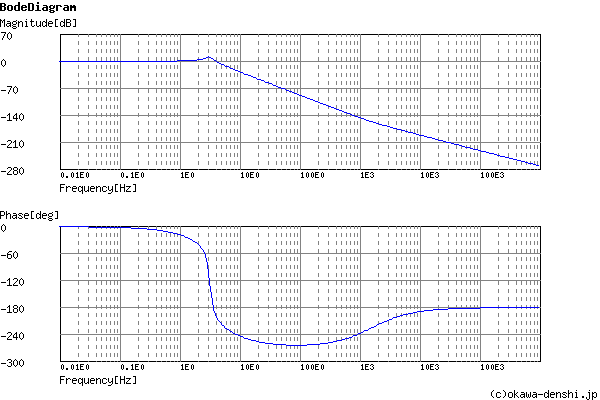Gain characteristics at the Bode Diagram (provides up to 1 minute)

Phase characteristics at the Bode Diagram (provides up to 1 minute)

Bode Diagram text data (provides up to 1 minute)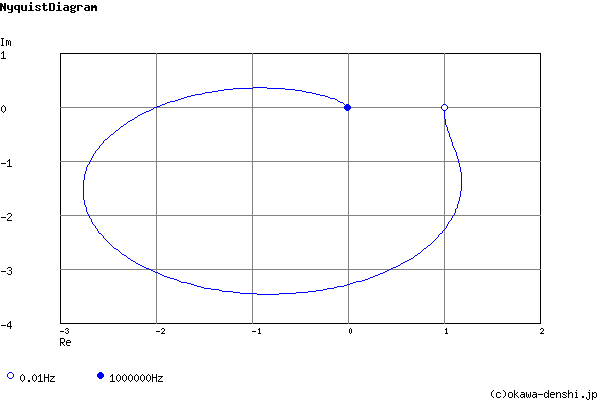Nyquist Diagram text data (provides up to 1 minute)

# Transient analysis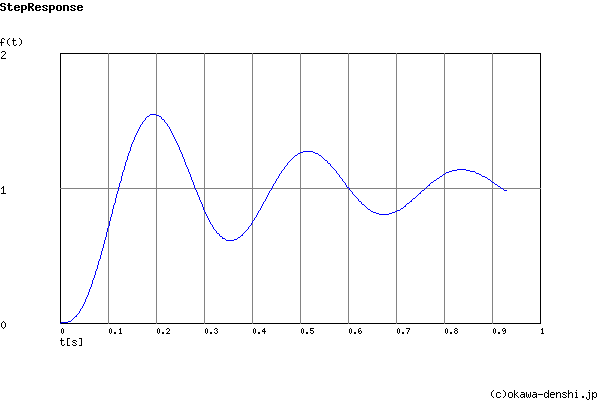Step Response text data (provides up to 1 minute)